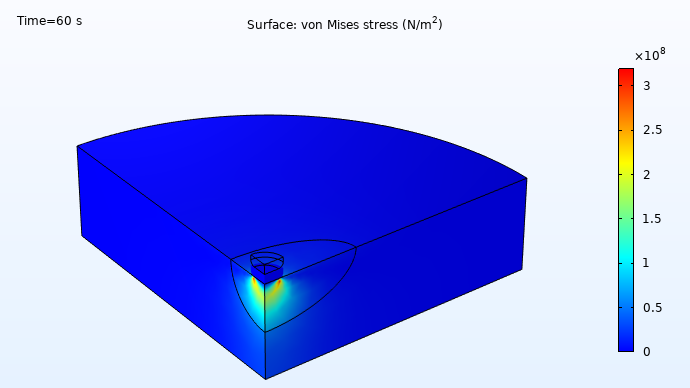# Learning Center Resources

Course:

Modeling Resistive and Capacitive Devices

## Modeling Resistive and Capacitive Devices

This 10-part, self-paced course is an introduction to modeling resistive and capacitive devices using COMSOL Multiphysics® and the AC/DC and MEMS modules. Through detailed demonstrations in the software, thorough explanations, and comprehensive discussions, you will learn how to build simulations of these types of devices. We start with learning the fundamentals of modeling resistors and capacitors separately. From there, we learn about meshing these types of devices, various ways we can refine the model to more efficiently simulate the device, defining and using nonlinear materials and their significance when modeling multiphysics, calculating capacitance matrices, and modeling electrostatics with a hybrid boundary element method and finite element method (BEM–FEM) approach.

From there, we learn about performing transient and frequency-domain analyses of devices with resistance and capacitance; modeling Joule heating under steady-state conditions, with nonlinear materials, in the time domain, and with time varying fields; introducing different types of feedback for controlling excitation, goal seeking, and more; and conclude with how to model parts moving relative to one another as well as into contact with each other.

An overview of the topics covered throughout the course is outlined below:A plot of the current density, show in arrows, and electric potential, visualized in a rainbow color table, for a resistor model.Simulation results showing the electric field dorm in a rainbow multislice plot.

Simulation results for models of a resistor (left) and capacitor (right) devices built in parts 2 and 3 of the course, respectively.

### Getting Started with Modeling Resistive and Capacitive Devices

##### Part 1: Fundamentals of Modeling Resistive and Capacitive Devices
• Set up your first model
• Compute the electric potential, electric currents, and device resistance
• Simplify the model
• Verify accuracy of the results
• Refine the mesh
##### Part 2: Resistors
• Focus the mesh refinement to a specific region of the model geometry
• Build models of a resistor
• Determine when to refine the mesh
• Introduce complexity to the model
• Determine what is and is not necessary to include in your model
##### Part 3: Capacitors
• Build models of a capacitor
• Model fringing fields
• Determine how much of the fringing fields should be included in your model
• Use infinite element domains in the model
• Introduce an inclusion to the model
##### Part 4: Capacitance Matrices
• Build models of capacitive systems with multiple electrodes
• Introduce the Stationary Source Sweep study and when to utilize it
• Introduce a dielectric to the model and move it around
• Perform a sensitivity analysis
• Model capacitance matrices in 2D and 3D using the BEM
##### Part 5: Electrostatics with a BEM-FEM Approach
• Compare use of FEM to BEM
• Discuss how to quantify computational cost of FEM versus BEM
• Introduce a hybrid FEM–BEM approach
• Introduce optimization into the model
• Use three different approaches to perform optimization on a capacitor model

### Modeling Considerations for Resistive and Capacitive Devices

##### Part 6: Transient and Frequency Domains
• Introduce a variation of the fields over time to the model
• Introduce a periodic variation of the fields over time to the model
• Use three different ways to calculate the cycle-averaged heating losses out of the system
• Convert between the time and frequency domains
• Discuss the relationship between the time and frequency domains
##### Part 7: Joule Heating with Nonlinear Materials
• Explain how the physics interfaces are coupled
• Postprocess results in various ways to visualize the Joule heating occurring in the device
• Introduce thermal boundary conditions
• Discuss tips and best practices for defining the physics for these types of models
• Change material properties so that they are nonlinear
##### Part 8: Joule Heating in the Time Domain
• Model a resistor and capacitor connected to a current source under steady-state conditions
• Compute resistance and capacitance of a device and show how to evaluate the time constant of the system
• Move model into the time domain and solve for conduction currents and displacement currents over time
• Use two different ways to introduce a variation into the simulation happening over time
• Discuss study options that can be used to compute the multiphysics model
##### Part 9: Introducing Feedback
• Explicit events and implicit events
• Voltage-limited terminals
• Specifying dissipated power
• Power terminal condition
• Implementing a PI controller
##### Part 10: Moving Domains
• Discuss how the geometry must be finalized to simulate movement
• Use features to ensure continuity of fields (electric currents, temperature, etc.)
• Verify the results for each physics interface
• Model moving parts
• Model moving parts with contactA model built in the last part of the course, where the moving domains are simulated. Here, an electrode comes into contact with and presses into an inclusion.

Model files are included in each part, which you can refer to as you follow along with the lecture in each part. Upon completing the course, you will gain a solid foundation in modeling resistors and capacitors. You will also be knowledgeable in the various tools, settings, features, and capabilities in the software that are available for you to use when creating such models.